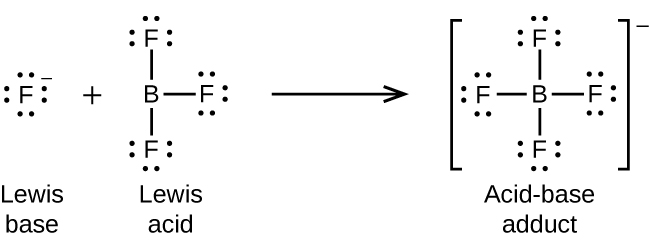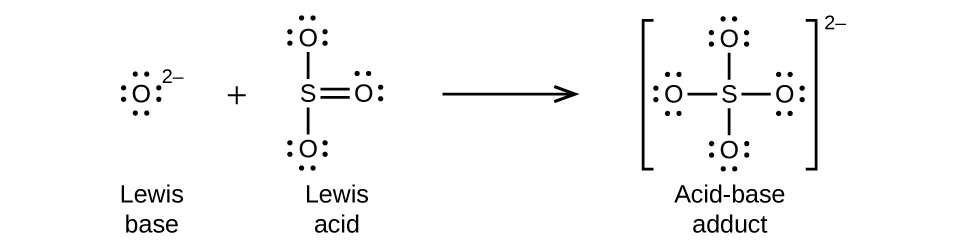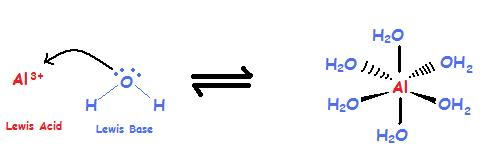# 16.7: Lewis Concept of Acids and Bases

$$\newcommand{\vecs}{\overset { \rightharpoonup} {\mathbf{#1}} }$$ $$\newcommand{\vecd}{\overset{-\!-\!\rightharpoonup}{\vphantom{a}\smash {#1}}}$$$$\newcommand{\id}{\mathrm{id}}$$ $$\newcommand{\Span}{\mathrm{span}}$$ $$\newcommand{\kernel}{\mathrm{null}\,}$$ $$\newcommand{\range}{\mathrm{range}\,}$$ $$\newcommand{\RealPart}{\mathrm{Re}}$$ $$\newcommand{\ImaginaryPart}{\mathrm{Im}}$$ $$\newcommand{\Argument}{\mathrm{Arg}}$$ $$\newcommand{\norm}{\| #1 \|}$$ $$\newcommand{\inner}{\langle #1, #2 \rangle}$$ $$\newcommand{\Span}{\mathrm{span}}$$ $$\newcommand{\id}{\mathrm{id}}$$ $$\newcommand{\Span}{\mathrm{span}}$$ $$\newcommand{\kernel}{\mathrm{null}\,}$$ $$\newcommand{\range}{\mathrm{range}\,}$$ $$\newcommand{\RealPart}{\mathrm{Re}}$$ $$\newcommand{\ImaginaryPart}{\mathrm{Im}}$$ $$\newcommand{\Argument}{\mathrm{Arg}}$$ $$\newcommand{\norm}{\| #1 \|}$$ $$\newcommand{\inner}{\langle #1, #2 \rangle}$$ $$\newcommand{\Span}{\mathrm{span}}$$$$\newcommand{\AA}{\unicode[.8,0]{x212B}}$$

## Introduction

Lewis Acid base theory is more general than the Bronsted-Lowery theory, and will be used extensively in organic chemistry. A Lewis acid is defined as an electron pair acceptor, and a Lewis base as an electron pair donor.Figure$$\PageIndex{1}$$: General schema for Lewis acid base reaction.

• Lewis Acid: a species that accepts an electron pair (i.e., an electrophile) and will have vacant orbitals, or the ability to form a vacant orbital.
• Lewis Base: a species that donates an electron pair (i.e., a nucleophile) and will have lone-pair electrons

In a Lewis Acid Base reaction a bond is formed where both electrons come from the Lewis base, and this is called a coordinate covalent bond (or dative covalent bond).

## Lewis Acids

There are three general types of Lewis Acids, that is, chemical species that can accept electrons.

1. Molecules with an incomplete octet
2. Molecules with double bonds
3. metal ions with vacant d-orbitals.

### Incomplete Octets

Boron Trifluoride is an exception to the octet rule and has an incomplete octet, and can thus accept electrons to form a bond. In the process it goes from sp2 hybrid to sp3 hybrid.Figure$$\PageIndex{2}$$: General reaction scheme where a molecule with an incomplete octet functions as a Lewis Acid

### Double Bonds

A double bond has 4 electrons shared between 2 atoms in a PI and sigma orbital. If you break the pi bond and move the two electrons to a non-bonding orbital on one atom, the other atom develops an incomplete octet and can function as a Lewis acid. This is shown in figure 16.7.3 where the pi electrons move onto the oxygen, allowing the sulfur to receive electrons from a Lewis base.Figure$$\PageIndex{3}$$: General reaction scheme where a molecule with a double bond functions as a Lewis Acid

### Metal Cations

Many metals have vacant d-orbitals that can accept electrons and thus behave as Lewis Acids. In fact, most metals react with water when they dissolve in water to form what is called a complex ion. For example, aluminum(III) is not a free ion, but a complex with 6 water molecules. Complex ions can be highly colored and will be covered in a later section of this text.Figure$$\PageIndex{4}$$: General reaction scheme where a molecule with a double bond functions as a Lewis Acid

## Lewis Bases

Lewis bases are molecules that have a lone pair of electrons that can be donated to a coordinate covalent bond. If you look at the Lewis dot structure of the bases in table 16.3.2 you will see that all of those bases have a nitrogen with a lone pair of electrons. This is the electron donor that forms the coordinate covalent bond. If the electron donor is a proton, then it is also a Bronsted base (proton acceptor).

## Lewis Acid Base Reactions

Lewis acid base reactions involve the formation of a coordinate covalent bond between the Lewis Acid (that accepts the electrons), and the Lewis base (that donates the electrons). Bronstead acid/base reactions are a subset of Lewis acid/base reactions where the coordinate covalent bond is formed with a proton accepting the electrons (Lewis Acid electron acceptor/ Bronstead Acid proton donor) from the base (Lewis Base electron donor/Bronstead proton acceptor). In the Bronstead acid/base theory a proton is transferred from the base to the acid, causing the charge to change of the subsequent conjugate acid and base, which in turn can form salts. Lewis acid base theory extends the concept of acid base reactions beyond this paradigm and is useful in predicting where bonds will form in many types of synthesis reactions. It should also be noted that the most primitive concept of acid/base reaction is the Arrhenius, which in itself is a subset of the Bronstead concept, involving the equilibrium of hydronium and hydroxide ion concentrations within water. So Lewis acid/base concept is the most general of the three concepts we use in describing acid base reactions.

The following animation should help make clear the types of Lewis acid/base reactions you are required to know and demonstrates how the Bronstead acid/base concept is a subset of the more general Lewis acid/base concept.

Video$$\PageIndex{1}$$: 1:31 YouTube uploaded by Douglas Mulford describing the above types of Lewis Acid base Reactions (https://youtu.be/6b3xELvF_ns).

Robert E. Belford (University of Arkansas Little Rock; Department of Chemistry). The breadth, depth and veracity of this work is the responsibility of Robert E. Belford, rebelford@ualr.edu. You should contact him if you have any concerns. This material has both original contributions, and content built upon prior contributions of the LibreTexts Community and other resources, including but not limited to:

This page titled 16.7: Lewis Concept of Acids and Bases is shared under a CC BY-NC-SA 4.0 license and was authored, remixed, and/or curated by Robert Belford.